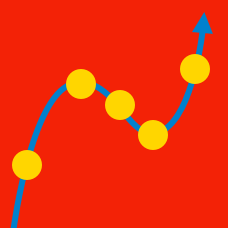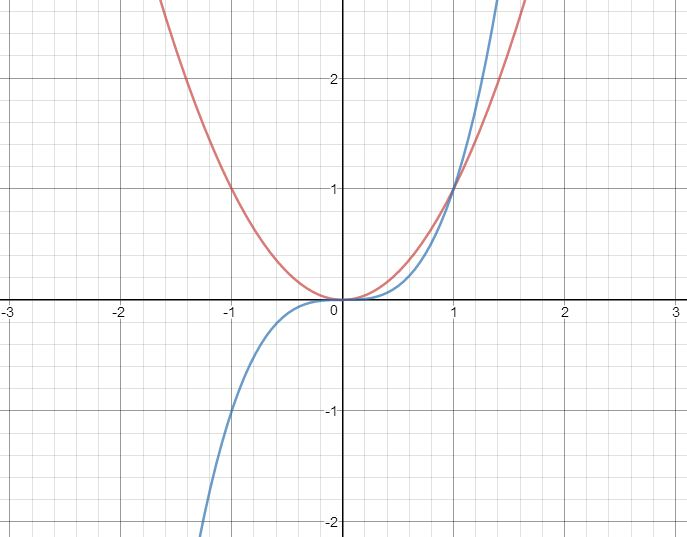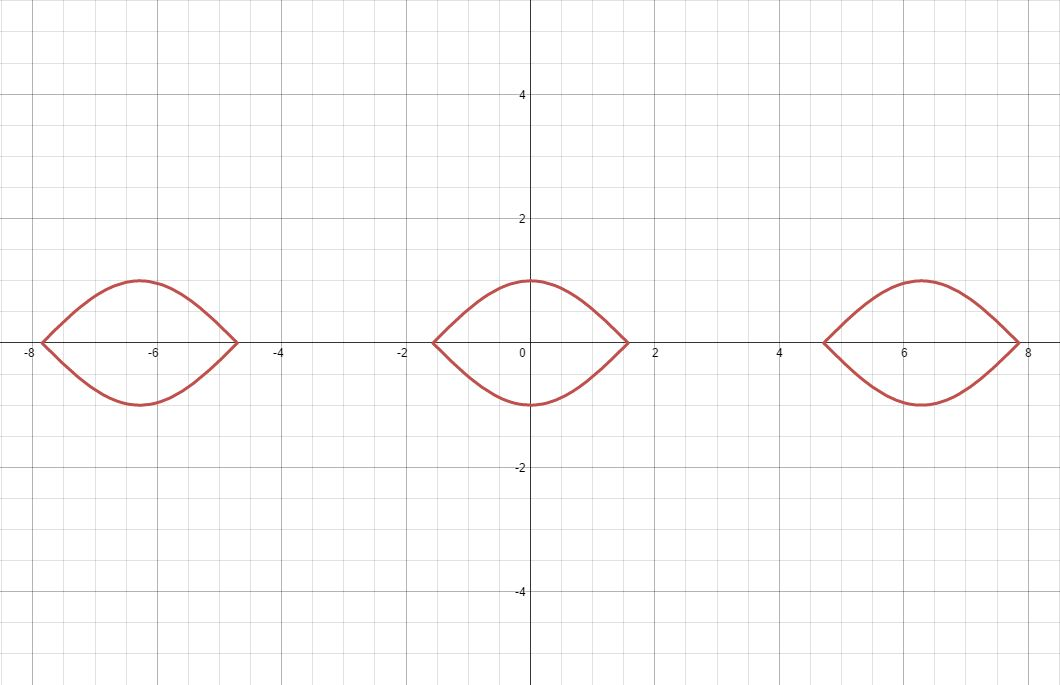Calculus

# Curve Sketching: Level 3 ChallengesThe above graph shows the curves of $x^2$ and $x^3$. Clearly, the line $y=0$ is tangent to both the curves. But there exists another such straight line which is tangent to both the curves. Find the equation of the line.

If the line can be represented in the form $ax+by+c=0$, such that $|a|,|b|$ and $|c|$ are positive integers and $\gcd(|a|,|b|,|c|) = 1$, enter your answer as $|a|+|b|+|c|$.

 Notation: $| \cdot |$ denotes the absolute value function.

If $f'(x)=|x|-\{ x \}$, then in which interval is the function $f(x)$ decreasing?

Details:

$\{ x \}$ represents sawtooth function. That is, $\{x\} = x - \lfloor x \rfloor$

Which of the given options does the graph represent?Notation: $| \cdot |$ denotes the absolute value function.

Let $f(x) = x^3 + ax^2 + bx + c$, where $a, b$, and $c$ are real numbers. In order for $f(x)$ to be invertible, $a$ and $b$ must be related as: $\dfrac{a^m}{b^n} \leq p$, where $m, n$, and $p$ are also real numbers.

Find the mimimum value of $m + n + p$.

$6 \ln (x^2 + 1) - x = 0$

How many real solutions exist for the equation above?

×